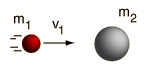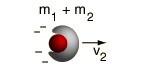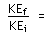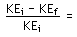# Inelastic Collision Calculation

Most collisions between objects involve the loss of some kinetic energy and are said to be inelastic. In the general case, the final velocities are not determinable from just the initial velocities. If you know the velocity of one object after the collision, you can determine the other (see inelastic head-on collisions). The extreme inelastic collision is one in which the colliding objects stick together after the collision, and this case may be analyzed in general terms.Initial data m1 = kg m2 = kg v1 = m/s Momentum = kg m/s Kinetic energy = JCalculated final values v2 = m/s Momentum = kg m/s Kinetic energy = J

Amount of kinetic energy lost in the collision = J.
 Ratio of kinetic energies before and after the collision:Fraction of kinetic energy lost in the collision:### Examples of inelastic collisions

Index

Collision concepts

 HyperPhysics***** Mechanics R Nave
Go Back